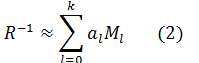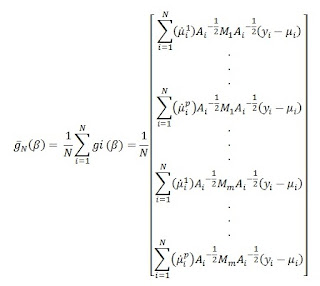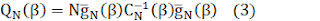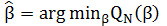Efficiency evaluation of quadratic inference functions in the analysis of longitudinal medical data

Year & Volume - Issue:
Authors:
Jamshid Yazdani-Charati, Maryam Tatari, Hamed Rouhanizade
Article type:
CID:
e0310
PDF File:romj-2018-0310.pdf
Abstract:
Background ― In medical research, longitudinal studies have important and functional roles. Generalized estimating equation (GEE) is used for the analysis of longitudinal and correlated data required to determine the correlation structure among responses. If this structure is set incorrectly, the parameter estimates will be consistent thus this method may not work. To improve the efficiency of parameter estimates, the quadratic inference function (QIF) method is therefore proposed. The objective of this study is to evaluate and compare the performance of these two methods. Methods ― This study was designed to compare the efficiency of parameter estimates using data from one blinded randomized clinical trial on the impact of probiotic drops on infantile colic. The effect of probiotic drops on crying time was modeled by GEE and QIF methods. Based on parameter estimates, the efficiency of the two methods was compared. Results ― The coefficient estimates of the two methods changed only slightly however, the relative efficiency of the parameter estimates from GEE and QIF was 1.23, when used on a mis-specified first-order autoregressive correlation structure. Therefore, for the specified correlation structure that is exchangeable, the relative efficiency was 1.001. The findings obtained from the QIF method showed that the mean baby crying had a significant difference on time between the two cases and control groups (P<0.001). Time (first, second and third weeks) was shown to be a major determinant of healing in infantile colic (P=0.001). Conclusion ― When selecting an incorrect correlation structure, the QIF method is more efficient than GEE. Thus, GEE can help researchers obtain more reliable results herein.
Cite as:
Yazdani-Charati J, Tatari M, Rouhanizade H. Efficiency evaluation of quadratic inference functions in the analysis of longitudinal medical data. Russian Open Medical Journal 2018; 7: e0310.

Introduction

To examine the impact of one or more independent variables on the response variable and the impact of different treatment methods in longitudinal studies  in medical science, psychiatry, biology and social sciences, response changes and factors affecting this change over time are determined. The characteristics of a longitudinal data set include repeated observations of the same variables in a data set. Therefore, changes in variables that lead to correlation are taken into account. In fact, the observation independence which is an assumption of conventional statistical methods is violated. Therefore the analysis of longitudinal data requires a particular statistical technique .

Important methodologies widely used to analyze longitudinal data, include marginal, mixed-effects and transition models. In this study, the marginal model is used for evaluation [1, 2].

Marginal models are a generalized estimating equation (GEE) approach proposed by Liang and Zeger in 1986. This method has gained considerable popularity in the analysis of longitudinal data in the past two decades .

The Quasi-likelihood method was developed for correlated data. This method does not require the joint distribution of a variable but only the moment and hypothetical correlation matrix which are used to avoid specification of the correlation matrix. This method assumes the estimation of the nuisance correlation parameter. Theoretically, if the correlation parameters are not properly estimated in these conditions, GEE may produce a consistent estimator but inefficiently [4-6].

Advances have been made to overcome some of the difficulties in the use of the GEE method. They include the quadratic inference function (QIF) approach proposed by Qu et al. in 2000 . This linear combination would be put in place of working correlation matrix in quasi-likelihood function and the generalized method of moments is used to obtain an objective function. The advantage of the QIF approach is that it can provide statistical inference of the regression parameters without requiring the estimation of the coefficients [5, 7-9].

Given the importance of efficient parameter estimation, this study compared two methods of estimation; QIF and GEE taking into account the correlation between correct and incorrect correlation structures using data from infantile colic.

Materials and Methods

Research data

This study was designed using statistical models on data obtained from a single-blind randomized clinical trial with no: "IRCT2016082829573N1". This study reports the impact of probiotic drop on the improvement of infantile colic in 98 infants referred to the Pediatric Gastroenterology Clinic in Sari (Iran) diagnosed with infantile colic. After explaining andobtaining the the parents’ consent, and using simple random sampling, the subjects were divided into groups of 49 patients each. In the intervention group, Biogaia probiotic drop was administered orally for 21 days and the control group received placebo during the same period. Probiotic drop volume was 5 cc administered as 5 drops daily. Parents of both groups were educated on breast feeding and accurate record keeping. Daily record of start and end times of crying in the night and day and the whole time crying in hours and minutes were recorded. The results of clinical evaluation on days 1, 7 and 21 of drug administration were examined. Daily crying time and doze of biogaia probiotic drop were analyzed weekly by a statistician.

Generalized estimating equations and quadratic inference function

For longitudinal data, let yit be an outcome variable and xit be a q×1 vector of covariates, corresponding to observations recorded at times t = 1, . . . , ni for subjects i = 1, . . . , N. Assume that the observations from different subjects are independent, but those within the same subject are dependent.

The marginal model relates the covariates to the marginal mean by the equation;

g(μi j )=x'i jβ,     (1)

where g is a known link function that depends on the type of response variable and β = (β0, β1, . . . , βq)' is a q-dimensional parameter vector .

In repeated vector measurements, the parameters of correlation are expressed in terms of the regression parameters separately. This correlation can be considered in the model by different structures:

• independent structure: it assumes that no correlation actually exists and observations within the series are independent.
• exchangeable structure: It assumes that there is a common correlation within observations.
• autoregressive structure: it is specified to set the within-subject correlations as an Exponential function of this lag period, which is determined by the user.
• unstructured structure: it posed no structure on the correlation matrix.

The GEE method finds the best fit by solving the score equation thus:wherewith Ai being the diagonal matrix of the marginal variances, μi and Ø is known as the dispersion parameter. Let Ri (α) be the working correlation matrix .

The QIF was derived by observing that the inverse of the working correlation matrix can be approximated by a linear combination of several basis matrices:,

where M0 is the identity matrix, M1, . . . ,Mk are known basis matrices with 0 or 1 as components and a0, . . . ,ak are unknown coefficients. Equation (2) holds exactly for some common working correlation structures. For example, if the working correlation is exchangeable, then R-1 = a0I + a1M1, where M1 is 0 on the diagonal and 1 elsewhere.

By substituting equations (2) in (1) leads to a linear combination of the elements of the following ḡN (β) extended score vector:As there are more equations than unknown parameters, the generalized method of moments can be applied by minimizing the QIF:whereis the sample covariance matrix [11, 12].

The QIF estimator is obtained with no need to estimate the nuisance correlation parameter:The objective function defined in equation (3) contains only the regression parameter β, and only the basis matrices from the working correlation structure are used to formulate this function. Hence, the QIF method does not rely on whether an appropriate estimation of the correlation parameter is available or not .

With the formula below, we can compare the efficiency of parameter estimates by GEE and QIF:If RE>1 thus QIF is more efficient than GEE; if RE<1 then GEE is more efficient than QIF and; if RE=1QIF, then GEE gives the same results [13, 14].

When the marginal model is used to fit the data, one primary tasks regarding model selection include the selection of a correlation structure. Akaike information criterion (AIC), Bayesian information criterion (BIC) and Q statistics are used for model selection. The model with the smallest QIF value was chosen as the simplest model with the best correlation structure [7, 14, 15].

Statistical analysis

Since the repeated measurements of baby cry create a correlated response, the analysis of this data requires procedures involving the correlation structure. In order to determine the effect of time variable and prescribed probiotics drops, on the marginal model, the QIF and GEE estimation procedures were applied using the SAS 9.3 software . P<0.05 was considered as statistically significant.

Results

A total of 98 patients were included in this study. They were divided into two groups; 49 patients each in the intervention and control groups. The general characteristics of the study participants are shown in Table 1. No significant differences were observed between the two groups with regards to the studied variables (Table 1).

Based on changes in the mean baby crying in the case and control groups, the greater reduction in the mean baby crying with time was seen in the intervention group as compared to the control. In fact, with the passage of time, mean baby crying declined in both groups, but further reduction was observed in the treatment group (Table 2).

The goodness-of-fit statistic from QIF aided in the optimal selection of correlation structure among several plausible choices. The exchangeable working correlation was better than first-order autoregressive correlation structure (AR-1) (Table 3). Results were obtained from fitting models in GEE and QIF methods for exchangeable and AR-1 correlationstructure (Tables 4 and 5).

Table 1. General characteristics of study participants

 Variables Intervention (n=49) Control (n=49) P-value Male gender 26 (51.2%) 24  (49%) 0.680a Natural childbirth 6 (24.5%) 12 (12.2%) 0.110a Dairy use 21 (42.9%) 27( 55.1%) 0.180a Weight, kg 3.28±1.15 3.15±1.41 0.490b Age, years old 36.12±1.65 29.32±1.07 0.051b Base crying mean 9.34±23.22 20.00±3.64 0.055b
Data presented as proportion (in percentage) – n (%), or mean with standard deviation – M±SD. a, Chi square; b, t-test.

Table 2. Crying mean changes over time in both intervention and control groups

 Variables Intervention (n=49) Control (n=49) Crying in 1 week 16.40±1.06 30.59±1.10 Crying in 2 week 11.36±1.93 24.19±1.81 Crying in 3 week 9.82±1.51 9.52±1.53
Data presented as mean with standard deviation, M±SD.

Table 3. QIF goodness-of-fit test for models 1 and 2

 Working correlation BIC AIC Q (P-value) AR 62 36 17 (0.070) Exchangeable 45 20 1 (0.001)

Table 4. Regression coefficient estimates based on GEE and QIF for exchangeable correlation

 Covariates GEE QIF Est. SE P Est. SE P Intercept -1.23 5.57 0.820 -1.23 5.57 0.820 Time -3.47 1.08 0.001 -3.47 1.08 0.001 Crying baseline 0.61 0.11 <0.001 0.61 0.11 <0.001 Use of drug (I/C) 8.60 3.58 0.015 8.64 3.58 0.010 Dairy use (y/n) -1.81 1.75 0.290 -1.81 1.75 0.290 Type of delivery (Ncb/Ces) -2.75 1.57 0.078 -2.75 1.57 0.070 Weight 0.002 0.005 <0.001 0.002 0.0005 <0.001 Age 0.19 0.12 0.107 0.19 0.12 0.100 Femal sex -0.83 1.52 0.580 -0.83 1.52 0.58 Time*druga 0.55 1.46 0.700 0.55 1.46 0.700

Est, Estimate; SE, standard errors; P, P-value; I/C, Intervention/Control; y/n, yes/no; Ncb/Ces, Natural child birth / Cesarean; a, interaction bethween time and drug.

Table 5. Regression coefficient estimates based on GEE and QIF for AR-1 correlation

 Covariates GEE QIF Est. SE P Est. SE P Intercept -2.3 5.39 0.660 -6.88 5.05 0.170 Time -3.47 1.08 0.001 -3.47 1.08 <0.001 Crying baseline 0.63 0.11 <0.001 0.75 0.09 <0.001 Use of drug (I/C) 8.50 3.54 0.016 4.25 2.33 0.060 Dairy use (y/n) -1.28 1.63 0.428 0.86 1.23 0.480 Type of delivery (Ncb/Ces) -2.27 1.53 0.137 -1.52 1.49 0.305 Weight 0.002 0.0005 0.003 0.002 0.0006 0.001 Age 0.17 0.11 0.104 0.07 0.04 0.030 Femal sex -0.65 1.43 0.640 0.56 1.13 0.610 Time*druga 0.55 1.46 0.700 2.10 1.03 0.030

Est, Estimate; SE, standard errors; P, P-value; I/C, Intervention/Control; y/n, yes/no; Ncb/Ces, Natural child birth / Cesarean; a, interaction bethween time and drug.

The efficiency of the two methods was compared using relative efficacy (RE), namely the difference of mean squared error obtained with exchangeable and AR-1 correlation structure.

In comparison to the efficiency of parameter estimates, the relative efficacy (RE) formula was used and the values 1.001 and 1.34 were obtained and used in the exchangeable and AR-1 correlation structure, respectively. This implied that the QIF parameter estimates were more efficient than the GEE Estimates while using the misspecified correlation structure.

When the working correlation structure is correctly specified, both the QIF and GEE are equally efficient. However, when the working correlation structure is misspecified, the QIF is more efficient than the GEE.

In order to analyze medical data, the QIF method with exchangeable working correlation structure was used.

The analysis showed that there was a statistically significant difference between the two groups for the mean baby crying (P<0.001). Thus, the mean baby crying in the group receiving probiotic drops was 8 hours less than the placebo group. The time variable was also significant as a factor in improving colic (P=0.001); the mean baby crying was reduced to 3.5 h with the passage of every week from the beginning of the intervention. The birth weight of infants and the mean baby crying at thebeginning of the study were also identified as contributing factors in improving infantile colic (P<0.05).

Discussion

In the present study, QIF and GEE methods were used to analyze longitudinal data set. Average infant crying over three consecutive weeks was compared in the intervention and control groups after the administration of probiotics drops.

The goodness-of-fit statistic from QIF also facilitates the optimal selection of correlation structure among several plausible choices. The results show that using the correct correlation structure, QIF and GEE estimators are the same, while an incorrect correlation structure uses the quadratic inference function mean square error of the less than generalized estimating equations. The QIF represents a more efficient method of inferential function which is secondary to the GEEs.

Songet et al. presented an introductory review of the QIF and in a simulation study found that:

• neither the AIC nor the BIC performed well for the selection of the correlation structure,
• there was a tendency that both criteriaover-select the exchangeable correlation over the AR-1 correlation,
• in practice, the true correlation is never known; therefore, the QIF appears to be more appealing as far as estimation efficiency is concerned .

Odueyungbo et al. evaluated GEE and QIF using data from the National Longitudinal Survey of Children and Youth (NLSCY) assuming AR-1 and exchangeable working correlation structures. They illustrated that the estimators from QIF were more efficient than GEE when the correlation structure is wrongly chosen . Abbadi et al. compared the two methods of GEE and QIF using longitudinal data from Bipolar I Disorder dataset in Mazandaran 2007-2011. This study showed that the estimates of the Quadratic Inference Function (QIF) method were more efficient than Generalized Estimating Equations method . Khajeh-Kazemi et al. utilized the use of QIF and GEE methods in comparing superior and inferior Ahmed glaucoma valve (AGV) implantation. Their focus was on the efficiency of estimation and using the model selection criteria. In the present study, QIF was more efficient than GEE. Therefore, in comparing GEE to QIF, the performance of the estimated parameters, despite consistency, was less efficient.

Smaller efficiency leads to misleading results in statistically significant independent variables . In Kun Yang et al.’s study, a total of 6,515 cases of data were enrolled to explore the association between platelet indices and blood pressure by QIF method. The GEE method was applied to make a comparison with QIF. QIF produced smaller standard errors compared with GEE in most situations even with the same working correlation matrix. This implied that parameters in the QIF model were more reliable and efficient than that of the GEE .

Using incorrect AR-1 correlation structures in the present study, an insignificant number of variables in the GEE were significant in QIF, and confidence intervals for regression coefficients of QIF were smaller than GEE. However, when using the correct exchangeable correlation structure, differences in statistical significance and confidence interval were not observed for each of the two methods. This confirms that QIF is more efficient.

Given that in the present study, the response variable was longitudinal, it is suggested to confirm the efficiency of QIF in correct and incorrect correlation structures. In addition, whether the response is binary or counting should be investigated.

Finally, analysis and interpretation of longitudinal data in the present study was performed using QIF with an exchangeable correlation structure.

From a clinical point and comparing with similar studies such as Jose Saavedra et al., long-term consumption of probiotic-containing milk powder is safe and provides sufficient microbial growth to reduce colic and irritability thus, less antibiotic use is required . In another study conducted by Savino and colleagues in Italy, it was shown that the use of probiotics can improve colic and reduce infants crying . Medical findings derived from this study are consistent with other studies and hence, can confirm the use of probiotic drops on the improvement of infantile colic.

Conclusion

When the correct correlation structure in the analysis of longitudinal data is selected, the parameter estimates are both relatively equal and consistent. However, when an incorrect correlation structure is used on QIF by GEE, the parameter estimates can be more efficient. When QIF is used by GEE, researchers are able to obtain reliable results. Due to the favorable properties of QIF, GEE can be applied as an alternative.

The results of this study show that the use of probiotics in the evolving gut can reduce infantile colic and improve the quality of life in infants. However, further studies on more variables are required to confirm these results.

Ethical approval

All procedures performed in studies involving human participants were in accordance with the ethical standards of the institutional and national research committee and with the 1964 Helsinki declaration and its later amendments or comparable ethical standards.

Acknowledgments

The present study was approved under the research code 683 in the School of Public Health, Mazandaran University of Medical Sciences (Sari, Iran). The data used in this research were registered in the Iranian Registry of Clinical Trials (IRCT201608 2829573N1; date of ethical approval: 8/2/2012) with the Code of Ethics Committee (1390-11-19).

Conflict of interest

The authors declare that they have no conflict of interest.

References:
1. Diggle P. Analysis of longitudinal data. Oxford University Press, 2002.
2. Fitzmaurice G, Davidian M, Verbeke G, Molenberghs G. Longitudinal data analysis. CRC Press, 2008.
3. Liang K-Y, Zeger SL. Longitudinal data analysis using generalized linear models. Biometrika 1986; 73(1): 13-22. https://doi.org/10.1093/biomet/73.1.13.
4. Crowder M. On consistency and inconsistency of estimating equations. Econometric Theory 1986; 2(3): 305-330. https://doi.org/10.1017/S0266466600011646.
5. Qu A, Lindsay BG, Li B. Improving generalised estimating equations using quadratic inference functions. Biometrika 2000; 87(4): 823-836. https://doi.org/10.1093/biomet/87.4.823
6. Tatari M, Yazdani Charati J, Karami H, Rouhanizadeh H. Effect of probiotics on infantile colic using the quadratic inference functions. Iranian Journal of Neonatology 2017; 8(3): 66-71. https://doi.org/10.22038/IJN.2017.9373.
7. Song PXK, Jiang Z, Park E, Qu A. Quadratic inference functions in marginal models for longitudinal data. Statistics in Medicine 2009; 28(29): 3683-3696. https://doi.org/10.1002/sim.3719.
8. Qu A, Song PXK. Assessing robustness of generalised estimating equations and quadratic inference functions. Biometrika 2004; 91(2): 447-459. https://doi.org/10.1093/biomet/91.2.447.
9. Khajeh-Kazemi R, Golestan B, Mohammad K, Mahmoudi M, Nedjat S, Pakravan M. Comparison of generalized estimating equations and quadratic inference functions in superior versus inferior ahmed glaucoma valve implantation. J Res Med Sci 2011; 16(3): 235-244. https://www.ncbi.nlm.nih.gov/pubmed/22091239.
10. Hedeker D, Gibbons RD. Longitudinal data analysis. John Wiley & Sons, 2006.
11. Song X-K, Song PX-K. Correlated data analysis: modeling, analytics, and applications. Springer Science & Business Media, 2007.
12. Han P, Song PX-K. A note on improving quadratic inference functions using a linear shrinkage approach. Statistics & Probability Letters 2011; 81(3): 438-445. https://doi.org/10.1016/j.spl.2010.12.010.
13. Casella G, Berger RL. Statistics Inference. Pacific Grove, California: Duxbury, 2002.
14. Qu A, Li R. Quadratic inference functions for varying‐coefficient models with longitudinal data. Biometrics 2006; 62(2): 379-391. https://doi.org/10.1111/j.1541-0420.2005.00490.x.
15. Gosho M. Criteria to select a working correlation structure for the generalized estimating equations method in SAS. J Stat Softw 2014; 57(1): 1-10. https://doi.org/10.1111/j.1541-0420.2005.00490.x.
16. Odueyungbo A, Browne D, Akhtar-Danesh N, Thabane L. Comparison of generalized estimating equations and quadratic inference functions using data from the National Longitudinal Survey of Children and Youth (NLSCY) database. BMC Med Res Methodol 2008; 8: 28. https://doi.org/10.1186/1471-2288-8-28.
17. Abadi A, Geraili Z, Yazdani J, Bakhtiari M, Saadat S. Comparison of Generalized Estimating Equations and Quadratic Inference Function in Longitudinal data of bipolar I disorder dataset in Mazandaran 2007-2011. Journal of North Khorasan University of Medical Sciences 2015; 7(4): 705-715. http://dx.doi.org/10.29252/jnkums.7.4.705.
18. Yang K, Tao L, Mahara G, Yan Y, Cao K, Liu X, et al. An association of platelet indices with blood pressure in Beijing adults: applying quadratic inference function for a longitudinal study. Medicine 2016; 95(39): e4964. https://doi.org/10.1097/MD.0000000000004964.
19. Saavedra JM, Abi-Hanna A, Moore N, Yolken RH. Long-term consumption of infant formulas containing live probiotic bacteria: tolerance and safety. Am J Clin Nutr 2004; 79(2): 261-267. https://doi.org/10.1093/ajcn/79.2.261.
20. Savino F, Pelle E, Palumeri E, Oggero R, Miniero R. Lactobacillus reuteri (American Type Culture Collection Strain 55730) versus simethicone in the treatment of infantile colic: a prospective randomized study. Pediatrics 2007; 119(1): e124-e130. https://doi.org/10.1542/peds.2006-1222

Jamshid Yazdani-Charati – Associate Professor of Biostatistics, Epidemiology and Biostatistics Department, School of Health, Health Science Research Center, Mazandran University of Medical Science, Sari, Iran.
Maryam Tatari – MS of Biostatistics, Department of Biostatistics, Student Research Committee, Mazandaran University of Medical Sciences, Sari, Iran; Faculty Member, Torbat Heydariyeh University of Medical Sciences,  Torbat Heydariyeh, Iran.
Hamed Rouhanizade – MD, Pediatrics, Pediatrics Department, School of Medicine, Mazandran University of Medical Science, Sari, Iran.

Received 1 November 2017, Revised 12 January 2018, Accepted 5 April 2018

© 2017, Yazdani-Charati J., Tatari M., Rouhanizade H.
© 2017, Russian Open Medical Journal

Correspondence to Maryam Tatari. E-mail: maryamtatary@yahoo.com. Phone: 00985152226013. Fax: 00985152226013.

DOI:
10.15275/rusomj.2018.0310
Author(s):